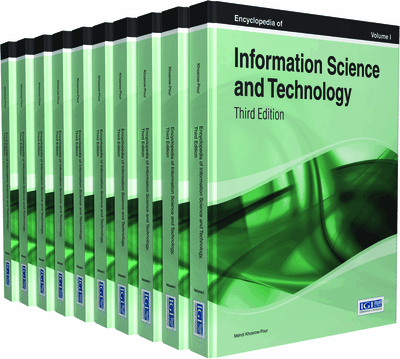# Machine Learning

Petr Berka (University of Economics, Prague, Czech Republic & University of Finance and Administration, Prague, Czech Republic)
DOI: 10.4018/978-1-4666-5888-2.ch020

Top

## Introduction

Machine learning was a founding branch of artificial intelligence in the late 1950s. However, it has become very attractive, especially recently, thanks to the Expert Systems (ES), Knowledge Discovery from Databases (KDD), Data Mining (DM), and their developments and applications. This article covers the following topics:

• Basic definitions of machine learning.

• Formal definition of empirical concept learning from data.

• Understanding learning as search or as approximation.

• Description of basic forms of selected learning algorithms.

Top

## Background

Human learning is one of the most important characteristics of human intelligence. In a similar way, machine learning (ML) is one of the most significant fields of artificial intelligence that attracts the interest of researchers for decades. Some researchers even argue that learning is so essential for intelligence, there can be no artificial intelligence without learning abilities.

There are numerous definitions of machine learning, most of them relating learning to the ability to improve a performance of a system over time:

The field of machine learning is concerned with the question of how to construct computer programs that automatically improve with experience. (Mitchell, 1997, p. XV)

Things learn when they change their behavior in a way that makes them perform better in the future. (Witten, 2005, p. 8)

Learning deals with “agents that can improve their behavior trough diligent study of their own experiences.” (Russell, 2009, p. 649)

We can distinguish two basic types of learning: concept learning and skill acquisition. Concept learning consists of inferring and assimilating new material composed of concepts, general laws, procedures, etc. The knowledge acquired should be expressed in a formalized description that allows us to solve a problem, explain a situation, predict behavior, etc. Refinement of skills through practice consists of gradually correcting deviations between observed and desired behavior through repeated practice. So an example of concept learning can be a learning process that results in describing the concept “dog” (as a hairy animal with four legs that barks) while an example of skill refinement can be learning to drive a car or play a piano.

Based on the feedback available during learning, we can distinguish different forms of learning. Reinforcement learning, where the teacher observes the learning system (typically a robot) and gives his feedback to the system in the form of rewards (when learning correctly) or punishments (when learning incorrectly), is the form of learning used for skill refinement. The other forms of learning referred here are used for concept learning:

• Supervised Learning: Where pre-classified examples are available for learning and the task is to build a (classification or prediction) model that will work on unseen examples,

• Unsupervised Learning: Where there is no feedback at all (this is typical for clustering and segmentation tasks)

• Semi-Supervised Learning: Falls between supervised and unsupervised learning; it uses a small number of pre-classified examples and a large number of examples without known class,

• Active Learning: Is a form of supervised learning, where the algorithm can query the teacher for class membership of unclassified examples,

• Apprenticeship Learning: Where the system uses indirect hints derived from the teacher´s behavior, is also a form of supervised learning.

We can also distinguish between analytic and empirical learning. In analytic learning the system formulates a generalization by observing only a single example (or absent any examples at all), and by exploiting an extensive background knowledge about the given domain. In empirical learning, the generalization is based on a huge amount of data (examples) and only very limited (if any) background knowledge.

## Key Terms in this Chapter

Artificial Neural Network: A representation of knowledge as a network of interconnected artificial neurons. The network expresses complex relationships between inputs and outputs, it can also be used to group together similar input examples.

Supervised Learning: A method of empirical concept learning from labeled data. The task is to build a classification or prediction model that assigns values of target attribute (class labels or values of numeric target) to previously unseen examples.

Semi-Supervised Learning: A method of empirical concept learning from both labeled and unlabeled data. A model created from a small amount of labeled data is used to classify unlabeled examples. These examples can then help during further learning improve the initial model.

Unsupervised Learning: A method of empirical concept learning from unlabeled data. The task is to build a model that finds groups of similar examples or that finds dependencies between attribute-value tuples.

Decision Rule: A representation of knowledge in an IF-THEN form usually used for classification. If the condition of the rule is satisfied, then the example belongs to the class given in the conclusion.

Association Rule: A representation of knowledge in an IF-THEN form. The association rule expresses the fact that transactions containing items from the condition part tend to contain transactions from the conclusion part.

Bayesian Network: A representation of knowledge in the form of a directed acyclic graph representing random variables as nodes and their conditional dependencies as edges.

SVM: A learning method where the classification knowledge has the form of a separating hyperplane.

Decision Tree: A representation of knowledge in a tree-like form usually used for classification. The non-terminal nodes of the tree represent questions, the terminal nodes represent class labels and the edges represent answers to questions.

## Complete Chapter List

Search this Book:
Reset